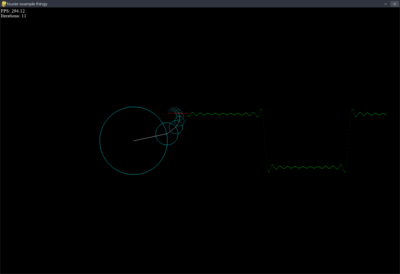# Fourier Series Demo - 1.0.0

A basic demo of the Fourier Series using pygame's unstable and sinful gfxdraw functionsNoorquacker
(Noorquacker)
A demo of the Fourier Series Approximation of a square wave function. Use the up and down arrow keys to change the amount of iterations in the approximation when in the program. It's pretty basic and unoptimized, read more about the Fourier Series at http://mathworld.wolfram.com/FourierSeries.html

### Changes

A demo of the Fourier Series Approximation of a square wave function. Use the up and down arrow keys to change the amount of iterations in the approximation when in the program. It's pretty basic and unoptimized, read more about the Fourier Series at http://mathworld.wolfram.com/FourierSeries.html